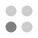• 0 trophies earned
• 0 Players Tracked
• 24 Total trophies
• 1,200 Points
• 0 Platinum Club
• 0 100% Club

### Pic-a-Pix Classic trophies

•Collect all the trophies0.00%
•Solve a 5x5 puzzle0.00%
•Solve a 10x10 puzzle0.00%
•Solve a 10x10 puzzle without using an X0.00%
•Solve all the 10x10 puzzles0.00%
•Earn a medal for every 10x10 puzzle0.00%
•Solve a 15x15 puzzle0.00%
•Solve a 15x15 puzzle without using an X0.00%
•Solve all the 15x15 puzzles0.00%
•Earn a medal for every 15x15 puzzle0.00%
•Solve a 20x15 puzzle0.00%
•Solve a 20x15 puzzle without using an X0.00%
•Solve all the 20x15 puzzles0.00%
•Earn a medal for every 20x15 puzzle0.00%
•Solve a 20x20 puzzle0.00%
•Solve a 20x20 puzzle without using an X0.00%
•Solve all the 20x20 puzzles0.00%
•Earn a medal for every 20x20 puzzle0.00%
•Solve a 30x20 puzzle0.00%
•Solve a 30x20 puzzle without using an X0.00%
•Solve all the 30x20 puzzles0.00%
•Earn a medal for every 30x20 puzzle0.00%
•Solve every puzzle0.00%
•Earn a medal for every puzzle0.00%